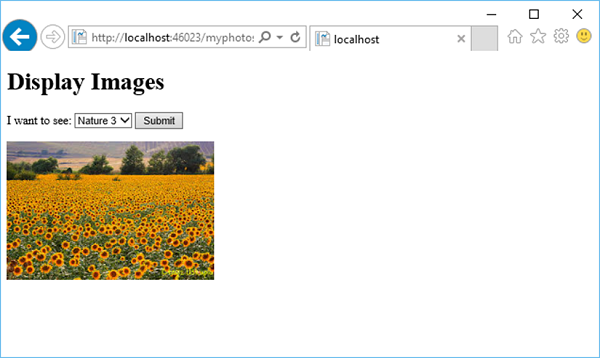# ASP.NET WP - Working with Forms

In this chapter, we will be covering how to create an input form and how to handle the user's input when you use the ASP.NET Web Pages using Razor syntax.

• A form is a section of an HTML document where you put user-input controls, like text boxes, check boxes, radio buttons, and pull-down lists.

• You use forms when you want to collect and process user input.

## How to Create an Input Form?

Let’s have a look into a simple example by creating a new cshtml file with MyForm.cshtml name and replace the code with the following program.

```<!DOCTYPE html>
<html>

<title>Customer Form</title>

<body>
<form method = "post" action = "">
<fieldset>
<div>
<label for = "StudentName">Student Name: </label>
<input type = "text" name = "StudentName" value = "" />
</div>

<div>
<label for = "UniName">University Name:</label>
<input type = "text" name = "UniName" value = "" />
</div>

<div>
<input type = "text" name = "Address" value = "" />
</div>

<div>
<label> </label>
<input type = "submit" value = "Submit" class = "submit" />
</div>
</fieldset>
</form>

</body>
</html>
```

Now let’s run the application again and specify the following url − http://localhost:46023/myform, then you will see the following output.Let’s enter some data in all the fields as shown in the following screenshot.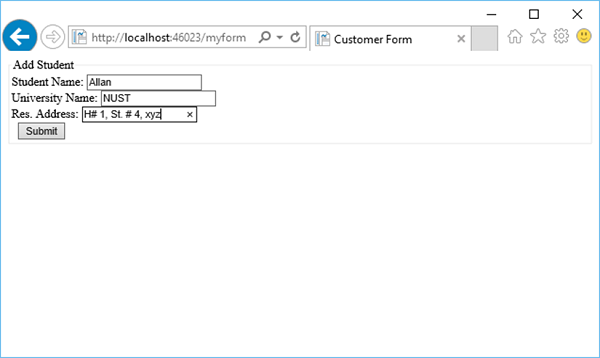Now, when you click the Submit button then you will see that nothing happens. To make the form useful, we need to add some code that will run on the server.

## Reading User Input from the Form

To read the user input from the form we will add some code that will read the values from all the fields and then process them as we want. This procedure shows you how to read the fields and display the user input on the page.

Let’s have a look into the same example again in which we have added some code that will handle all the values from the fields.

```<!DOCTYPE html>
<html>

<title>Customer Form</title>

<body>
@{
if (IsPost){
string StudentName = Request["StudentName"];
string UniName = Request["UniName"];

<p>
You entered: <br />
Student Name: @StudentName <br />
University Name: @UniName <br />
</p>
} else{
<form method = "post" action = "">
<fieldset>
<div>
<label for = y"StudentName">Student Name: </label>
<input type = "text" name = "StudentName" value = "" />
</div>

<div>
<label for = "UniName">University Name:</label>
<input type = "text" name = "UniName" value = "" />
</div>

<div>
<input type = "text" name="Address" value = "" />
</div>

<div>
<label> </label>
<input type = "submit" value = "Submit" class = "submit" />
</div>

</fieldset>
</form>
}
}
</body>
</html>
```

Now let’s run the application again and specify the following url − http://localhost:46023/myform then you will see the following output.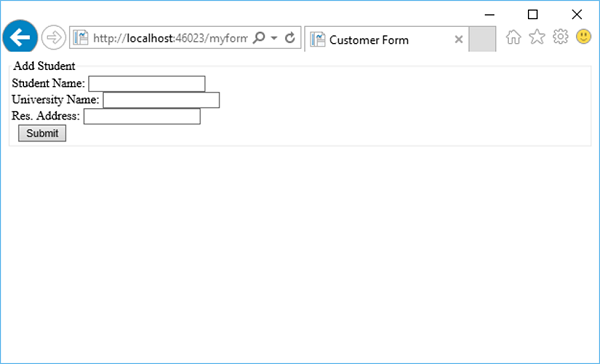Let’s enter some data in all the fields.Now when you click the Submit button then you will see the following output.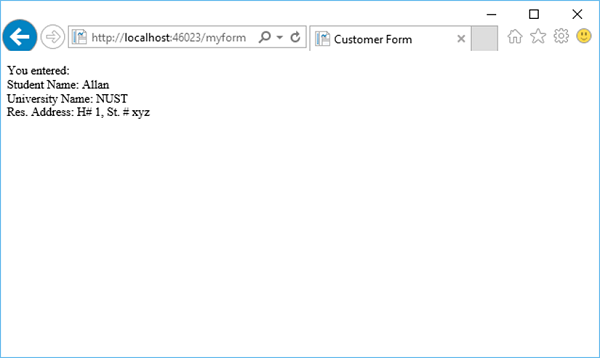Let’s have a look into another simple example by creating a new folder in your project and by naming it as images and then add some images in that folder.

Now add another cshtml file with MyPhotos.cshtml name and replace the following code.

```@{
var imagePath = "";

if (Request["Choice"] != null)
{ imagePath = "images/" + Request["Choice"]; }
}

<!DOCTYPE html>
<html>

<body>
<h1>Display Images</h1>

<form method = "post" action = "">
I want to see:
<select name = "Choice">
<option value = "index.jpg">Nature 1</option>
<option value = "index1.jpg">Nature 2</option>
<option value = "index2.jpg">Nature 3</option>
</select>
<input type = "submit" value = "Submit" />

@if (imagePath != ""){
<p><img src = "@imagePath" alt = "Sample" /></p>
}
</form>

</body>
</html>
```

As you can see that we have added references of some jpg files which are in the images folder as shown in the following screenshot.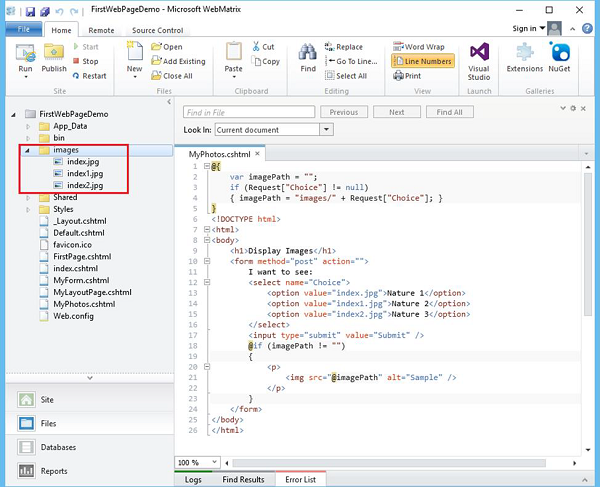When you run the application and specify the following url − http://localhost:46023/myphotos then you will see the following output.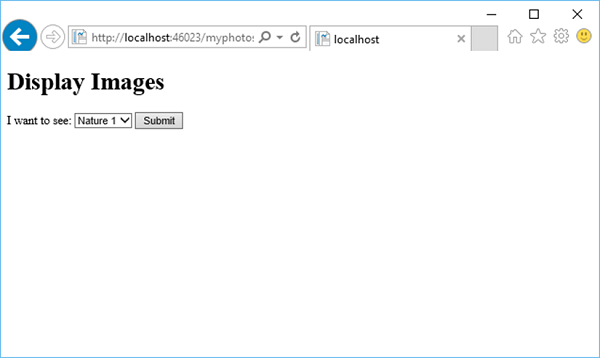Let’s click on Submit and you will see that the index.jpg file is loaded on the page.When selecting another photo from the dropdown list, let’s say Nature 3 and click Submit, then it will update the photo on the page as shown in the following image.